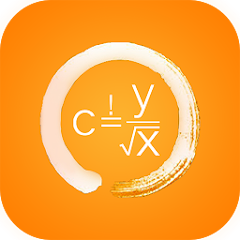# Physics?MPP!

50+Everyone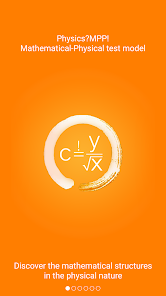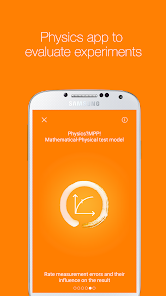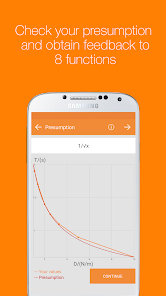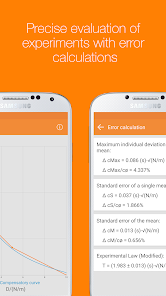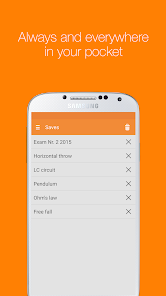Version for pupils \$ 2,99 (incl.tablet version)

Suitable for young people aged 15 and over, pupils and students, for use in the experimental teaching, practical training, exam preparation, high school preparation, baseline and apprenticeship.
Includes scientific error analysis and forecasting capabilities.

How does a formula come into the physics book?

The investigation of physical issues, resulting to appropriate experiments, leads to a list of a series of measurements.
The evaluation of these measurements will be made with the mathematical-physical test model, that is the basis of the app "Physics?MPP!" and the connection of the two pillars of physics, the experiment and mathematics.

As a result of investigation of appropriate proportionalities, there is appearing, in conjunction with a precise measurement, an experimental law. This shows - generalized - the way to the physical formula.

Furthermore the user gets feedback on three key questions:

Can you achieve the 10% criterion?
School experiments with series of measurements are successful, if the measurement error is less than 10%. Physics?MPP! helps you evaluate either your own experiment or a given measurement session.

Can you recognize the formula?
Successful experiments with measurements lead to a quantitative relationship between the measured values by an experimental law. If you choose the correct guess in the graphical representation, you'll receive an experimental law, that shows the way to the physical formula.

You can calculate values with the experimental law, which can be verified in your experiment by measurement. You can evaluate the reliability and validity of this physical measurement too.

Further objectives are:
- Discover the mathematical structures in the physical nature
- Compute experimental results and check them
- Rate measurement errors and their influence on the result
- Recognize the physical meaning of a constant

Physics?MPP! shows:
- How quantitative relationships between physical quantities result with physical experiments
- How with a constant the quality of a measurement can be assessed
- How predictions can be made with an experimental law
- How careful planning and implementation of a experiment can be demonstrated with an error calculation
- How by mental considerations new physical quantities and fundamental constants are defined
- How through generalization of experimental contexts formulas enter into the physics book

The inspiration of Physics?MPP! was a simple question of a pupil: "How does a formula enter into the physics book?"

Physics?MPP! is based on the fundamental conviction: In every child is an explorer! (... and in every youth or adult is still a child).

In my years as a teacher I had the idea to unite the two pillars of physics, the math and the experiments in a model. The result is the mathematical-physical test model, that shows the way to a physical formula on the basis of an experimental law and is the basis of Physics?MPP! too.
Updated on
Mar 6, 2017

## Data safety